# Download Excel Spreadsheet Templates for Watershed Time of Concentration Calculation

## Time of Concentration in Hydrologic Calculations

The time of concentration of a watershed is the time that it takes for rainfall landing on the hydrologically farthest point of the watershed to reach its outlet. The watershed time of concentration is of interest primarily because of its use as the storm duration for determination of the design storm rainfall intensity to use for rational method calculation of peak storm water runoff rate.

The time of concentration is used for this design storm duration because it will give the maximum peak storm water runoff rate for a given recurrence interval. The rationale is as follows: For storm durations less than the time of concentration, the storm will end before runoff from the entire watershed is reaching the outlet, so the entire watershed will never be contributing flow at the outlet. For storm durations greater than the time of concentration, the storm will continue beyond the time when the entire watershed starts to contribute flow at the outlet, but a longer duration storm will have a lower intensity than a shorter duration storm with the same recurrence interval. Thus a storm of duration equal to the time of concentration will indeed give the maximum peak storm water runoff rate for a specified recurrence interval.

Ok, so that’s why there is interest in the time of concentration, now read on for information on how to calculate values for the time of concentration of a given watershed.

## Methods of Estimating Time of Concentration

Many different empirical equations have been developed for calculation of travel time and time of concentration for the many variations in types of watersheds. These include the Izzard equation, the Kerby equation, the Kirpich equation, the Manning Kinematic equation, the National Resources Conservation Service (NRCS) method, the Bransby Williams equation, and the Manning equation.

For this article, three of the equations will be discussed. The Manning Kinematic equation will be presented for use with overland sheet flow, the NRCS method will be presented for shallow concentrated flow, and the Manning equation will be discussed for channel flow. These three methods of calculating travel time are those recommended by the U.S. Soil Conservation Service (SCS) in the first reference at the end of this article. These methods are also given in the second reference, the Iowa Stormwater Management Manual.

## Excel Spreadsheet Templates for Travel Time by the Manning Kinematic Equation

The Manning Kinematic equation is shown in the boxes at the right for U.S. and for S.I. units.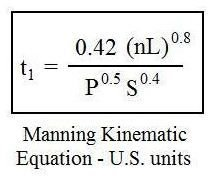The parameters in the equation are as follows:

• t1 = overland sheet flow runoff travel time, min (NOTE: many places show the constant being 0.007 for U.S. units giving the time in hours. These equations both give travel time in minutes.)
• n = Manning roughness coefficient, dimensionless
• L = length of the flow path, ft (S.I. – m)
• P = 2 year, 24 hr rainfall depth, in (S.I. – mm)
• S = ground slope, ft/ft (S.I. – m/m)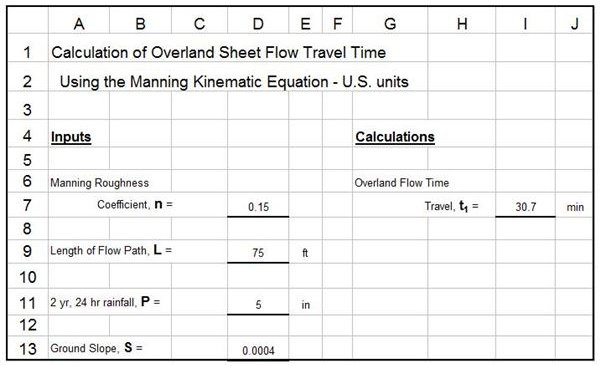The Excel spreadsheet template image shown at the left is set up to calculate the overland sheet flow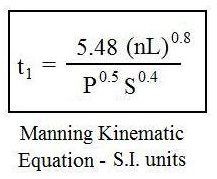travel time using the Manning kinematic equation, based on the input value entered for the other parameters listed above. Tables with values of the Manning roughness coefficient for various overland flow conditions are available in all three of the references for this article.

Click here to download a table of n values prepared from information in Reference #2.

Additional sections of an overall spreadsheet for calculation of watershed time of concentration are presented and discussed separately in the next few sections. U.S. and S.I. versions of the entire spreadsheet can be downloaded from links at the end of this article, on page 2.

## Excel Spreadsheet Templates for Travel Time by NCRS Method

Reference #1 for this article recommends using the Manning Kinematic equation for travel length of no more than 300 ft and reference #2 recommends that L should be no more than 100 ft for that equation. Both of these methods recommend using the NCRS method for the shallow concentrated flow that typically develops within 100 to 300 ft into the watershed. The approach used in the NCRS method is to first calculate the velocity of the shallow concentrated flow based on the type of surface and slope. Then the travel time is calculated by dividing the travel length by the velocity of flow. The NCRS method equations are as follows:

• t2 = L/(60V) ( for either U.S. or S.I. units )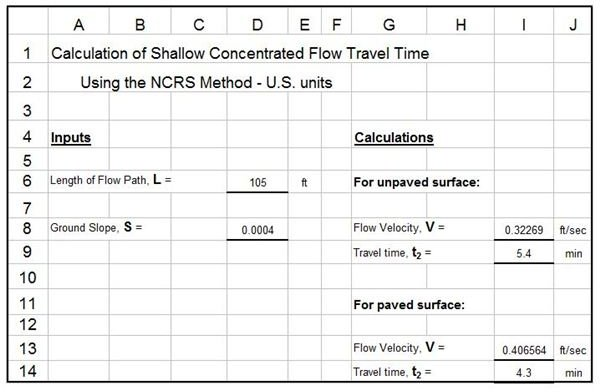• V = 16.1345 S0.5 for U.S. units ( V = 4.9178 S0.5 for S.I. units) for an unpaved surface
• V = 20.3282 S0.5 for U.S. units ( V = 6.1960 S0.5 for S.I. units) for a paved surface

The parameters in these equations and their units are as follows:

• t2 is the travel time for shallow concentrated flow in minutes (for either U.S. or S.I. units)
• L is the length of the flow path in ft for U.S. or m for S.I. units
• V is the velocity of flow in ft/sec for U.S. or m/s for S.I. units
• S is the slope of the flow path, which is dimensionless for either U.S. or S.I. units

A Excel spreadsheet template that will calculate the shallow concentrated flow travel time using the NCRS method is shown at the right above. It is part of the overall time of concentration Excel spreadsheets that can be downloaded through links at the end of this article.

## Excel Spreadsheet Templates for Travel Time by The Manning Equation

The Manning Equation is widely used for open channel flow calculations and is recommended in both of the first two references to calculate the travel time for any channel flow portion of the watershed runoff path. The equations used in this part of the spreadsheet are as follows:

• The Manning equation in U.S. units: Q = (1.49/n)A(R2/3)(S1/2)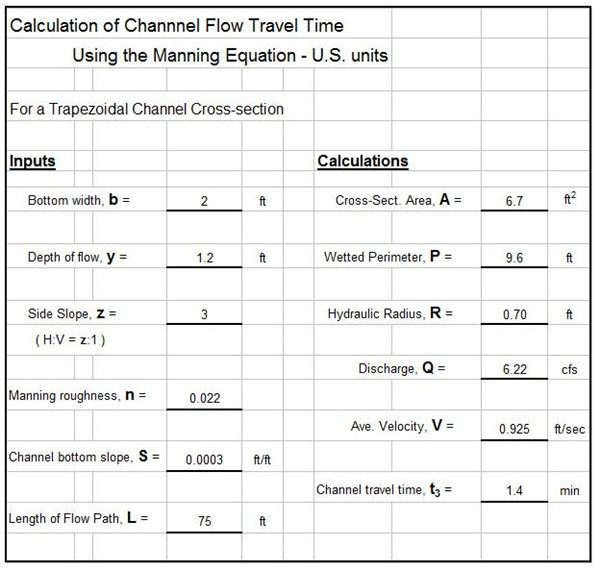• The Manning equation in S.I. units: Q = (1.0/n)A(R2/3)(S1/2)
• R = A/P
• V = Q/A
• t3 = L/(60V)

The parameters in these equations and their units are as follows:

• Q = channel flow rate in cfs for U.S. units or m3/s for S.I. units
• V = average velocity of flow in ft/sec for U.S. units or m/s for S.I. units
• R = hydraulic radius of the channel (= A/P) in ft for U.S. units or m for S.I. units
• A = channel cross-sectional area in ft2 for U.S. units or m2 for S.I. units
• P = wetted perimeter of channel in ft for U.S. units or m for S.I. units
• S = channel bottom slope, which is dimension for either set of units
• n = Manning roughness coefficient for channel
• L = length of flow path in ft for U.S. units or m for S.I. units
• t3 = travel time for channel flow in min for either set of units

An image of an Excel spreadsheet to calculate channel travel time using the Manning equation is shown at the upper right. This is part of the overall Excel spreadsheets for watershed time of concentration that can be downloaded in U.S. or S.I. units from links in the next section. For additional background on Manning equation calculations and more downloadable Excel spreadsheets, see the article, "Use of Excel Spreadsheet Formulas for Uniform Open Channel Flow/Manning Equation Calculations."

## Overall Excel Spreadsheets for Calculating Time of Concentration

The overall watershed time of concentration is simply the sum of all the travel times calculated as described in the last three sections. An overall Excel spreadsheet template containing the modules described above for Manning kinematic equation, the NCRS method, and the Manning equation calculations, along with provision for summing all of the travel times to get the watershed time of concentration, can be downloaded in U.S. or S.I. units by clicking on the appropriate link below.

## References

References for Further Information:

1. U.S. Soil Conservation Service, Technical Note – Hydrology No N4, June 17, 1986.

2. Iowa Stormwater Management Manual, Section on Time of Concentration.

3. Knox County Tennessee Stormwater Management Manual, section on the Rational Method.

4.Bengtson, Harlan H., Hydraulic Design of Storm Sewers, Including the Use of Excel, an online, continuing education course for PDH credit.

## This post is part of the series: The Rational Method for Calculating Peak Storm Water Runoff Rate

The Rational Method is widely used to calculate the peak storm water runoff rate for a variety of storm water management applications. The drainage area, runoff coefficient, and design rainfall intensity are needed for the storm water runoff calculation.